# Adversarial Neural Cryptography in Theano

Last week I read Abadi and Andersen’s recent paper , Learning to Protect Communications with Adversarial Neural Cryptography. I thought the idea seemed pretty cool and that it wouldn’t be too tricky to implement, and would also serve as an ideal project to learn a bit more Theano. This post describes the paper, my implementation, and the results.

## The setup

The authors set up their experiment as follows. We have three neural networks, named Alice, Bob, and Eve. Alice wishes to communicate an N bit message P to Bob. Alice and Bob also share a key (which you can think of as a password) of N bits.

Alice takes the message and the key, and encrypts the message, producing a communication C of N bits. Bob receives this communication, and then attempts to decrypt it, producing PBob.

Unfortunately for Bob and Alice, Eve intercepts Alice’s communication C. She then decrypts this message herself, producing her attempted recovery of P, which is called PEve.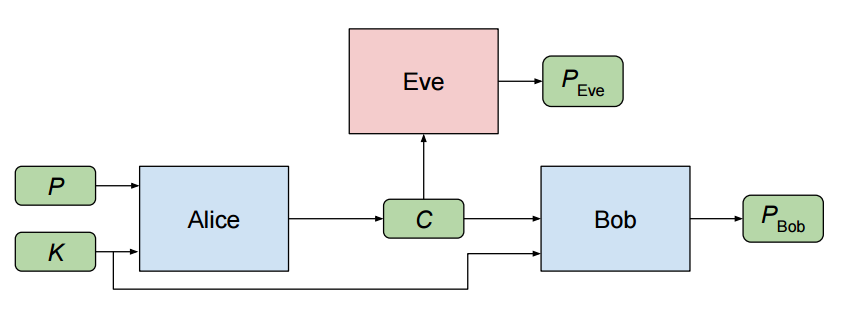Figure 1: The adversarial network setup diagram given in .

### Neural networks

As mentioned, Alice, Bob and Eve are all neural networks. All three of these networks are quite similar.

Alice (Figure 2) takes as input the message and key vectors, concatenated into one long vector of length 2N. This then passes through a single fully-connected hidden layer of size 2N. It then passes through what I will refer to as the standard convolutional setup, which takes this 2N-length vector, passes it through a number of 1D convolution filters, and eventually outputs an N-length vector. This is the communication C that gets sent to Bob.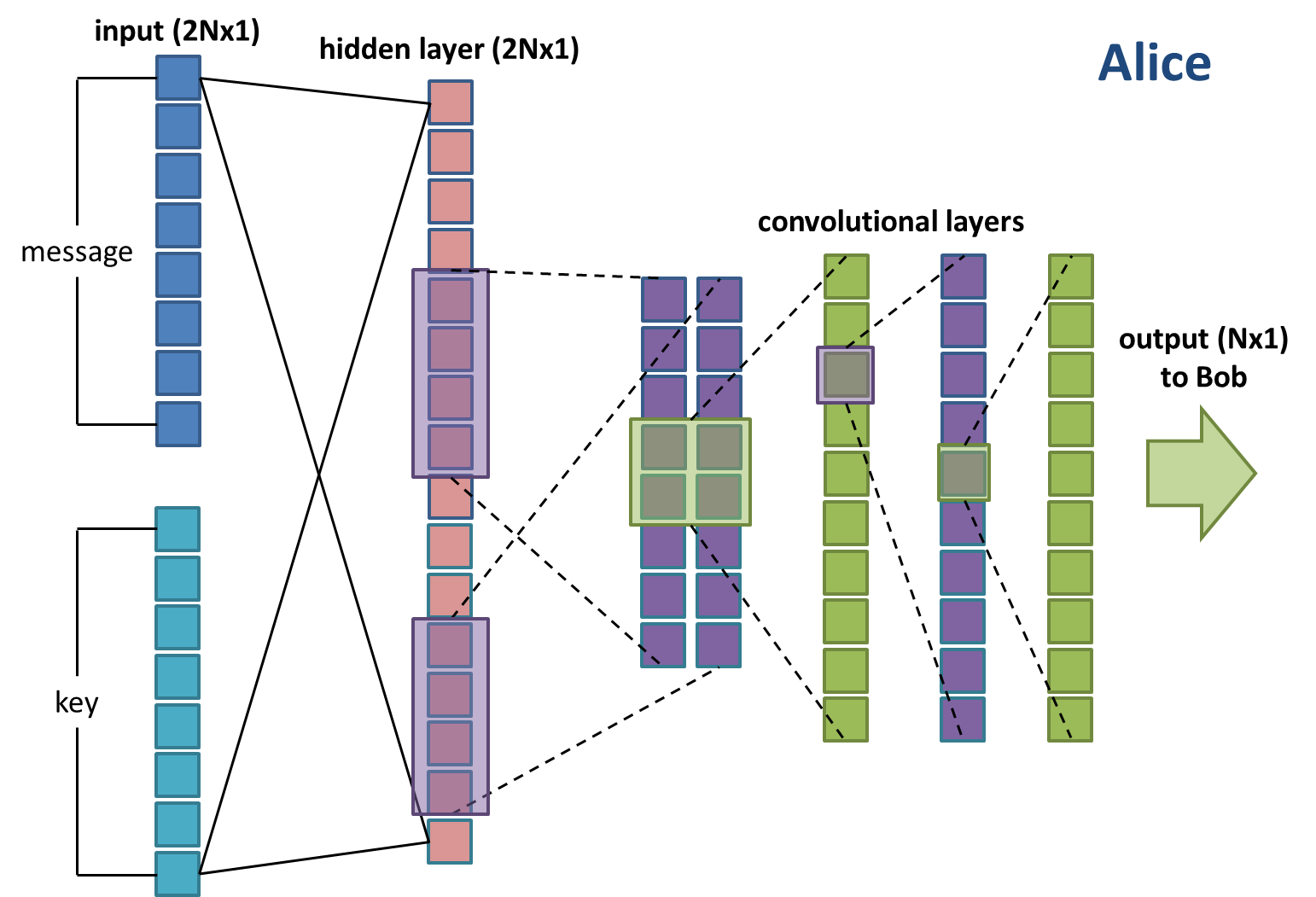Figure 2: Alice’s neural network setup.

Bob’s network (Figure 3) is identical to Alice’s, except that his input is the concatenation of the communication and the key.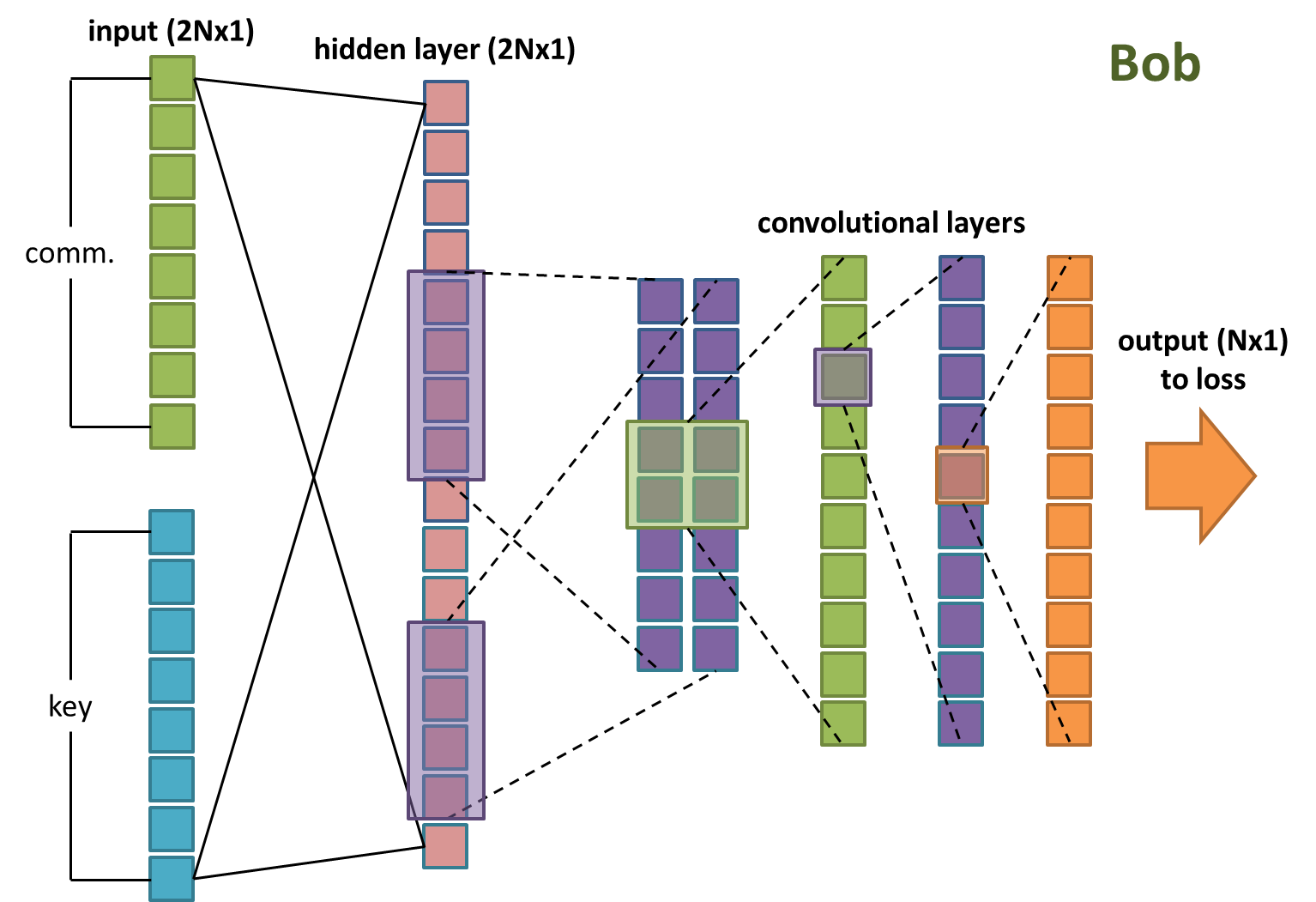Figure 3: Bob’s neural network setup.

Eve’s network is also quite similar to Bob and Alice’s. However her input is just the communication C. She also has an additional fully-connected hidden layer of size 2N prior to the standard convolutional setup: the authors wanted to make Eve a bit more complex in order to give her a better chance of figuring out how to decrypt C.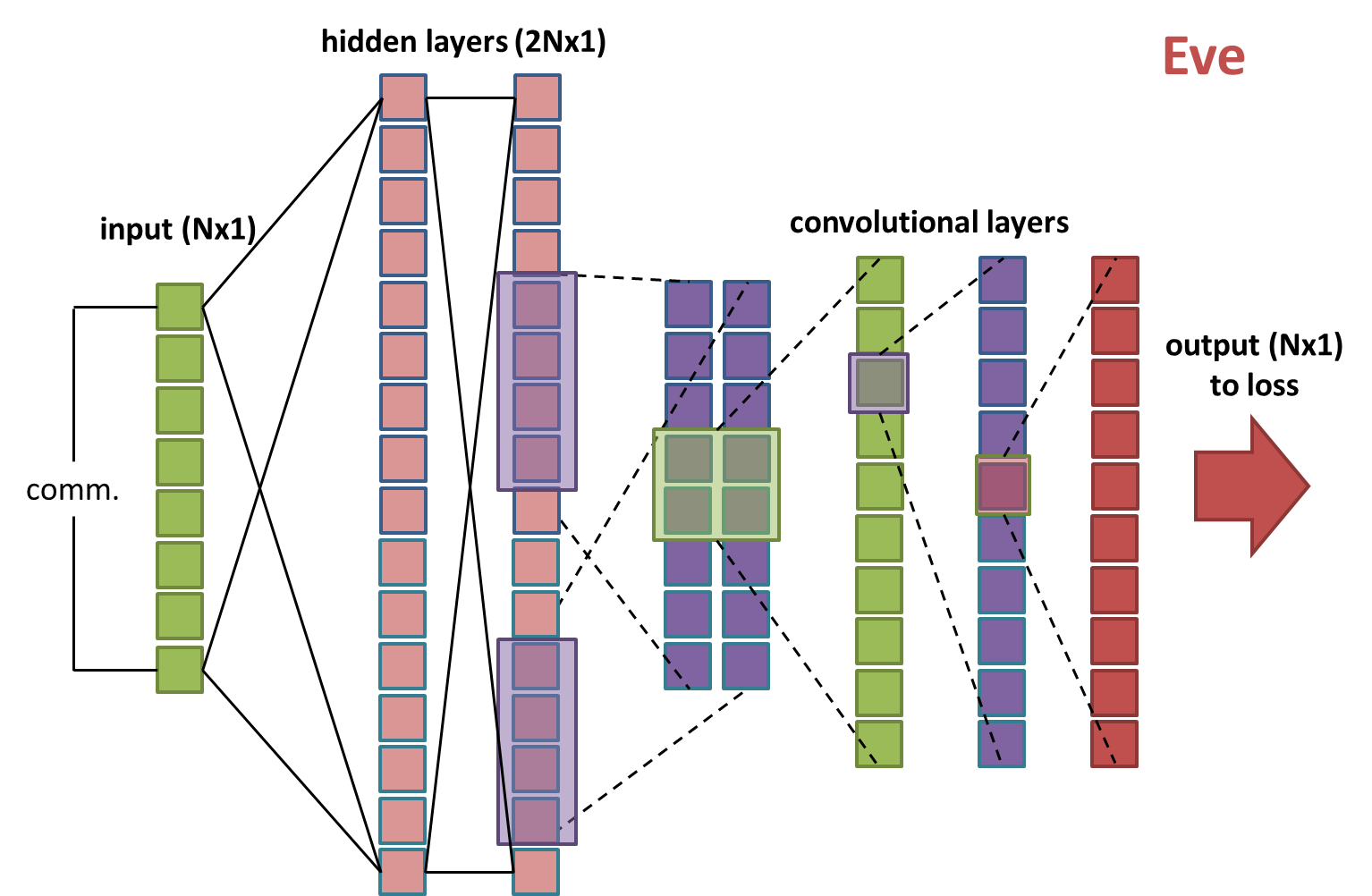Figure 4: Eve’s neural network setup.

The following table gives the output dimensionality of each layer in each network:

Layer Output shape
Alice input 2N
Alice hidden 2N
Alice convolutional (2, 2N+1) –> (4, N) –> (4, N) –> (1, N)
Bob input 2N
Bob hidden 2N
Bob convolutional As per Alice
Eve hidden 1 2N
Eve hidden 2 2N
Eve convolutional As per Alice

### Loss functions

The next ingredient are the loss functions:

Eve’s loss function is simply the absolute difference between the message vector P and her attempt at decrypting it PEve. This loss is backpropagated through the weights in the Eve network to update her weights.

LossEve = Eve L1 error = abs(P - PEve)

Bob and Alice’s loss function is also the absolute decryption error, plus an additional term that signifies how well Eve is currently decrypting the message. This additional term is:

(N / 2 - Eve L1 error)2 / (N / 2)2

This is minimised when Eve gets half the bits correct, on average, in her decryption, which indicates that she is doing no better than random guessing. The gradient of this loss function is used to update the weights in both the Bob and Alice networks. Putting these together, we have:

LossBob = Bob L1 error + (N / 2 - Eve L1 error)2 / (N / 2)

Training then proceeds in an adversarial way: we train Bob and Alice for a while until they are communicating effectively, then we train Eve for some time, until she learns to decrypt the message. Then we train Bob and Alice again, who figure out how to fool Eve’s current decryption method. Then we train Eve again, who cracks the improved encryption, and so on. The authors find that after a while, Bob and Alice’s encryption becomes too strong for Eve to be able to learn to crack it.

## Theano implementation

The implementation is fairly straightforward. I have used some custom classes, HiddenLayer() and ConvLayer() for adding standard NN layers. You can find these in the layers.py file.

We first need to define our batch size and N up front. I have added the possibility to have different lengths for the key, message and communication, however I have not tested this; changing it might cause issues.

### Alice and Bob

For Alice and Bob we just create a fairly straightforward sequential NN:

### Eve

Eve is similarly implemented. We just need to use alice_comm in her inputs.

### Loss functions

Here we just implement the loss equations described in the previous section. Note that the additional term in Bob’s loss function is a bit simpler than the equation described above. Things have been set up such that a mean error of 1 means that half the bits were correctly decrypted (as bits are input as either -1 or 1, so a single error = 2). Hence the N/2 terms can be dropped from the implementation.

### Training functions

The only tricky-ish thing here is making sure that the training function for Alice and Bob updates all their parameters, while Eve’s only updates her parameters. I use lasagne.adam for an implementation of the Adam SGD optimiser. I put the functions in dictionaries for ease of use in adversarial training.

### Convolution layers

Since it is used in all three networks, I made a custom class for the standard convolutional setup. It stores all the parameters and tensors relavent to all of the convolutional layers in the model. I have tried to match the description of the convolution setup described in the paper:

### Training

To perform the adversarial training, I made a train() function that would train either Alice and Bob or Eve for some time. We then just iterate between calling this function on Alice and Bob, and then for Eve. The gen_data() function generates batch_size random message and key pairs. We train according to the loss, but for plotting we just store the decryption error for the party that is currently being trained.

## Results

I trained both Alice and Bob, and then Eve, for up to 2000 iterations at a time (early stopping occurred if the decryption error was below 0.01 for a while). I did 60 overall repetitions of this adversarial training setup. I then plotted the minimum decryption error achieved by Bob and by Eve in each of these 60 runs (Figure 5).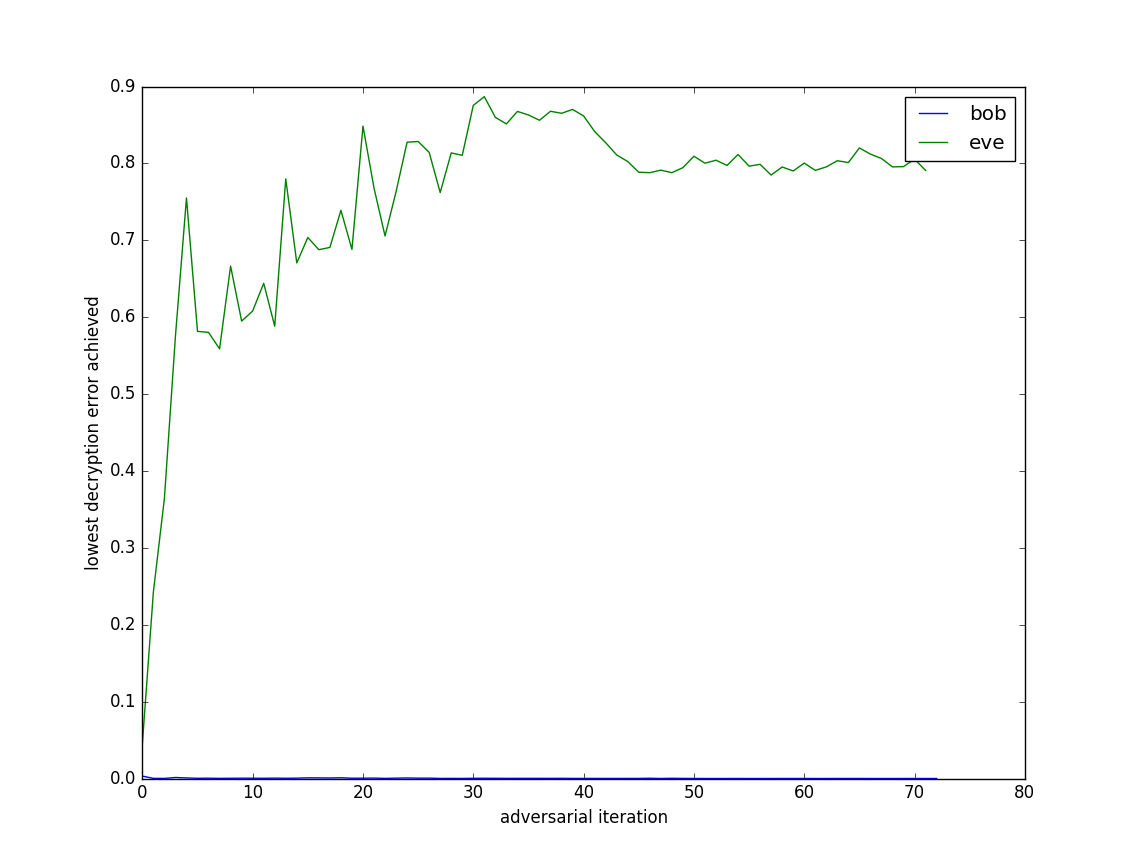Figure 5: Bob and Eve’s decryption errors over 60 adversarial training iterations.

So, it seems to work. After a few adversarial rounds, Bob and Alice figure out a way to effectively scramble the communication such that Eve cannot learn how to decrypt it.

I also tested the setup without the four convolutional layers, instead replacing this with an additional 2N in, 1N out hidden layer (Figure 6).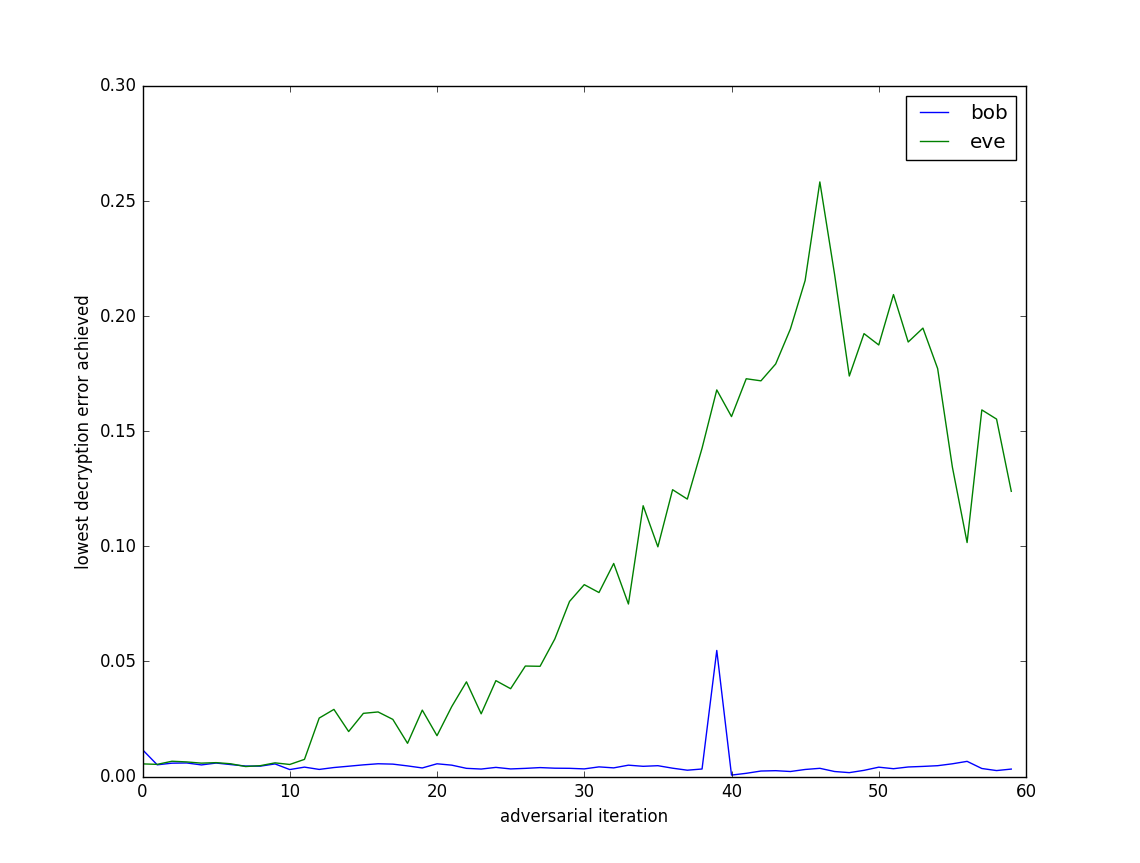Figure 6: Bob and Eve’s decryption errors over 60 adversarial training iterations, with the convolutional phase of the network excluded.

This seems to suggest that the convolution layers helps, but perhaps it is still possible to achieve the goals of this experiment without it - Eve still isn’t able to perfectly recover the message in this setup either.

## Final thoughts

I should note that this paper didn’t receive much love when it was posted on the Reddit MachineLearning forum. And I have to say I kind of agree with the points made in that discussion: really the fact that this works doesn’t mean it has created good encryption. Rather it more just speaks to the weakness of the Eve network in its ability to decrypt the message. This is sort of reflected by the fact that this setup still seems to work without the convolution layers (Figure 6). Still, it is an interesting idea, and I don’t think I’m in a position to judge its academic merit.